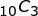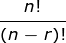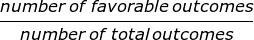# Probability Flashcards

Probability Flashcards
1/23 (missed) 0 0
Create Your Account To Continue Studying

As a member, you'll also get unlimited access to over 79,000 lessons in math, English, science, history, and more. Plus, get practice tests, quizzes, and personalized coaching to help you succeed.

Try it risk-free for 30 days. Cancel anytime
Among 100 people, 12 have blonde hair, 50 have red hair, 28 have brown hair, and 10 have black hair. If two people are selected at random, what is the probability that both will have red hair?

49/198

Got it
I flip a coin and draw a card from a standard deck of 52 cards. What's the probability that I get heads on the coin and draw an ace?

1/26

Got it
If you draw two cards at random from a standard deck of 52 cards (and don't replace the first one), what is the probability of drawing two spades?

156/2652 = 3/51 = 1/17

The probability of the first spade is 13/52.

The probability of drawing a second spade after not replacing the first is 12/51.

13/52 * (12/51) = 156/2652 = 3/51 = 1/17

Got it
If set A = {2, 4, 6, 8, 10, 12} and set B = {3, 6, 9, 12}, find the intersection of A and B.

{6, 12}

Got it
If set A = {2, 4, 6, 8, 10, 12} and set B = {3, 6, 9, 12}, find the union of A and B.

{2, 3, 4, 6, 8, 9, 10, 12}

Got it
Calculate the following:120

Got it

1/5

Got it
What is the probability that the sum of two die will be less than seven given that the first die rolled was a two?

2/3

Got it
You're packing for vacation. You have 2 red shirts, 3 green shirts, 5 blue shirts, and 1 white shirt. If the first shirt you packed was red, what is the probability that the second shirt is red?

1/10

Got it
You want to read ten books. How many different ways can you select the first five books you read if the order in which you read them is important?

30240

Got it
A governing presidency is being formed from among 8 people. Three people will be in this presidency: a president, a vice president, and a secretary. How many ways can such a presidency be formed?

336

Got it

or choose a specific lesson: See all lessons in this chapter
23 cards in set

## Flashcard Content Overview

This cards will give you practice problems on the principles of probability. In these flashcards, you'll encounter permutations and combinations, independent and dependent events, and review some basic ideas of set theory.

Front
Back
A governing presidency is being formed from among 8 people. Three people will be in this presidency: a president, a vice president, and a secretary. How many ways can such a presidency be formed?

336

You want to read ten books. How many different ways can you select the first five books you read if the order in which you read them is important?

30240

You're packing for vacation. You have 2 red shirts, 3 green shirts, 5 blue shirts, and 1 white shirt. If the first shirt you packed was red, what is the probability that the second shirt is red?

1/10

What is the probability that the sum of two die will be less than seven given that the first die rolled was a two?

2/3

1/5

Calculate the following:120

If set A = {2, 4, 6, 8, 10, 12} and set B = {3, 6, 9, 12}, find the union of A and B.

{2, 3, 4, 6, 8, 9, 10, 12}

If set A = {2, 4, 6, 8, 10, 12} and set B = {3, 6, 9, 12}, find the intersection of A and B.

{6, 12}

If you draw two cards at random from a standard deck of 52 cards (and don't replace the first one), what is the probability of drawing two spades?

156/2652 = 3/51 = 1/17

The probability of the first spade is 13/52.

The probability of drawing a second spade after not replacing the first is 12/51.

13/52 * (12/51) = 156/2652 = 3/51 = 1/17

I flip a coin and draw a card from a standard deck of 52 cards. What's the probability that I get heads on the coin and draw an ace?

1/26

Among 100 people, 12 have blonde hair, 50 have red hair, 28 have brown hair, and 10 have black hair. If two people are selected at random, what is the probability that both will have red hair?

49/198

If I roll a die and get a three, what is the probability of rolling a three the second time I roll the die?

1/6

A coin is flipped twice. What is the probability of getting one heads and one tails?

1/2

When drawing two cards at the same time from a standard deck of 52 cards, what is the probability of drawing a 10 and an ace?

2/13

The probability of a 10 is 4/52 or 1/13.

The probability of an ace is also 4/52 or 1/13.

These are mutually exclusive. Add these together and you get 2/13.

When two dice are rolled, what is the probability of rolling a sum of 10?

1/12

On a d8, or 8-sided die, each side shows a number from 1-8. If you roll two d8s, what is the probability that the sum of the numbers will be 7?

3/32

This is an example where you use the number of favorable outcomes over the total number of possible outcomes. Favorable outcomes for this event include

dice 1 : dice 2

1 : 6

2 : 5

3 : 4

4 : 3

5 : 2

6 : 1

So, there are 6 favorable outcomes.

There are 8 possible outcomes for dice 1 and 8 possible outcomes for dice 2. This gives us a total of 64 possible outcomes (8*8)

Favorable outcomes / Total outcomes = 6 / 64 = 3 / 32

Three dice are rolled. What is the probability that at least one of them shows a 6?

91/216

First, we can calculate the probability of not rolling a six on any of the three dice. The probability of not rolling a six on one die is 5/6. So, the probability of not rolling a six on three dice is 5/6 * 5/6 * 5/6 or (5/6)^3 = 125/ 216.

Now, this is the probability of not rolling a six on any die. However, we want to know the probability of rolling a six on at least one of them. This is equal to 1 - (125/216) = 91/216

In a group of 100 women, 12 have blonde hair, 50 have red hair, 28 have brown hair, and 10 have black hair. If one person is selected, what is the probability she has red or brown hair?

39/50

On a d20, or 20-sided die, each side shows a number from 1-20. If I roll one three times in a row, what's the probability of rolling a 10 three times in a row?

1/8000

Give the formula to find a permutation of n objects where r are selected.

Permutation formula:Formula for the probability of a single eventHow to find the probability of compound events

Multiply the probabilities together

How do we find P(A U B) if A and B are mutually exclusive?

P(A U B) = P(A) + P(B)

To unlock this flashcard set you must be a Study.com Member.